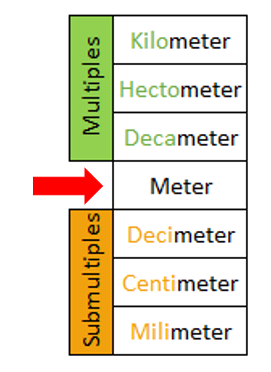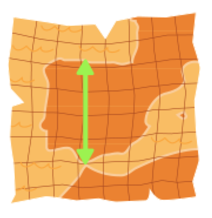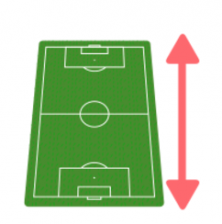Smartick is an online platform for children to master math in only 15 minutes a day

Jan30

This week we will address the topic of measurements of length. Do you know what they are? Do you know what they are used for? We will explain it to you in this post.

The length determines the distance between two points, or in other words, it is the amount of space between two points.  For example, the distance between my house and school, or the distance from one end of the table to the other.

The principal unit for measuring length is the meter. For example, a meter is what a guitar measures in length.1 meter

But, what do I do if I want to measure much smaller objects? Or much bigger objects?

For that, we have more measurements of length: multiples and submultiples of the meter.

• Multiples are the units of measurements which are bigger than the meter. They are the decameter, hectometer, and the kilometer. There are more, but right now we are only going to look at these.
• Submultiples are the units of measurement which are smaller than the meter. They are the decimeter, the centimeter, and the millimeter.

Shown in the table below are the main measurements of length for the metric system:In order for you to have a rough idea of the distances that multiples and submultiples measure, let’s look at the following examples:The distance between Florida and North Carolina is approximately 900 kilometers.The length of a soccer field is approximately 1 hectometer.The length of a bus is approximately 1 decameter.The height of a bottle of water is approximately 2 decimeters.The length of a tennis ball is approximately 6 centimeters.

I hope you liked this week’s post and that you have learned the measurements of length. If so, don’t hesitate to share it with your friends and classmates so that they can learn, too.

And remember that you can learn and practice measurements of length and all the elementary math topics on Smartick in an effective and fun way. Register for free and try it without any commitment.